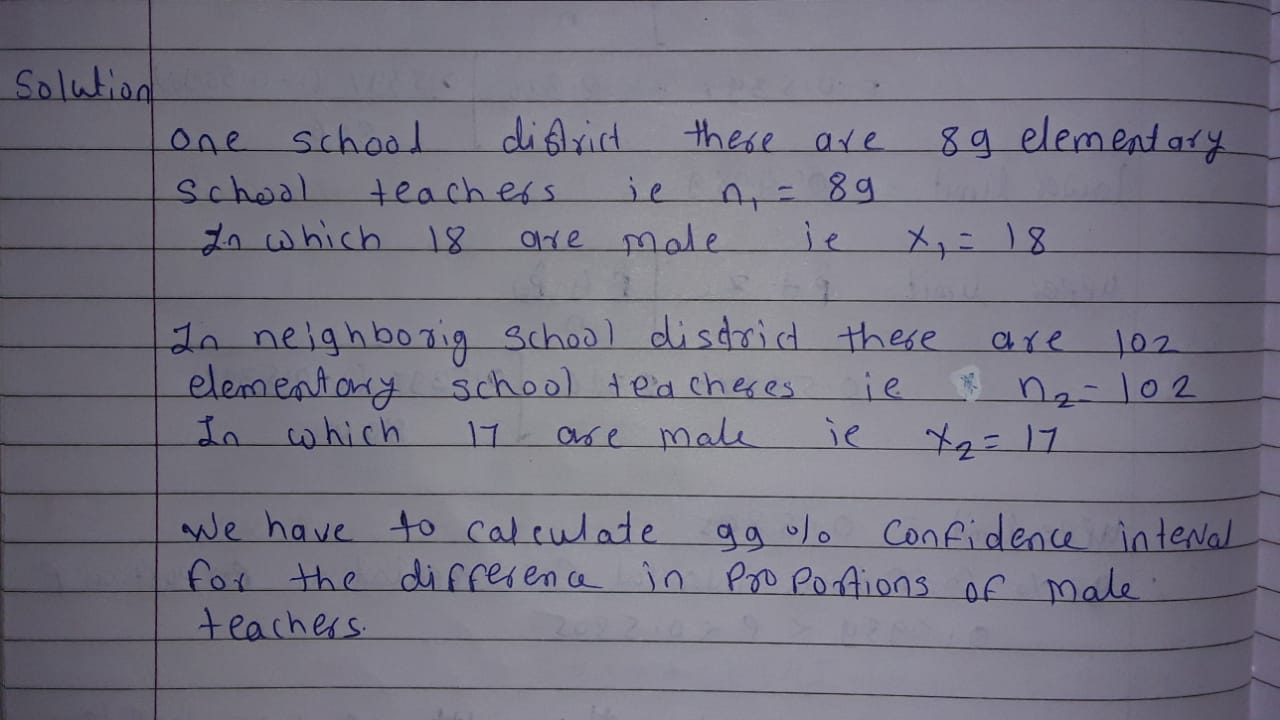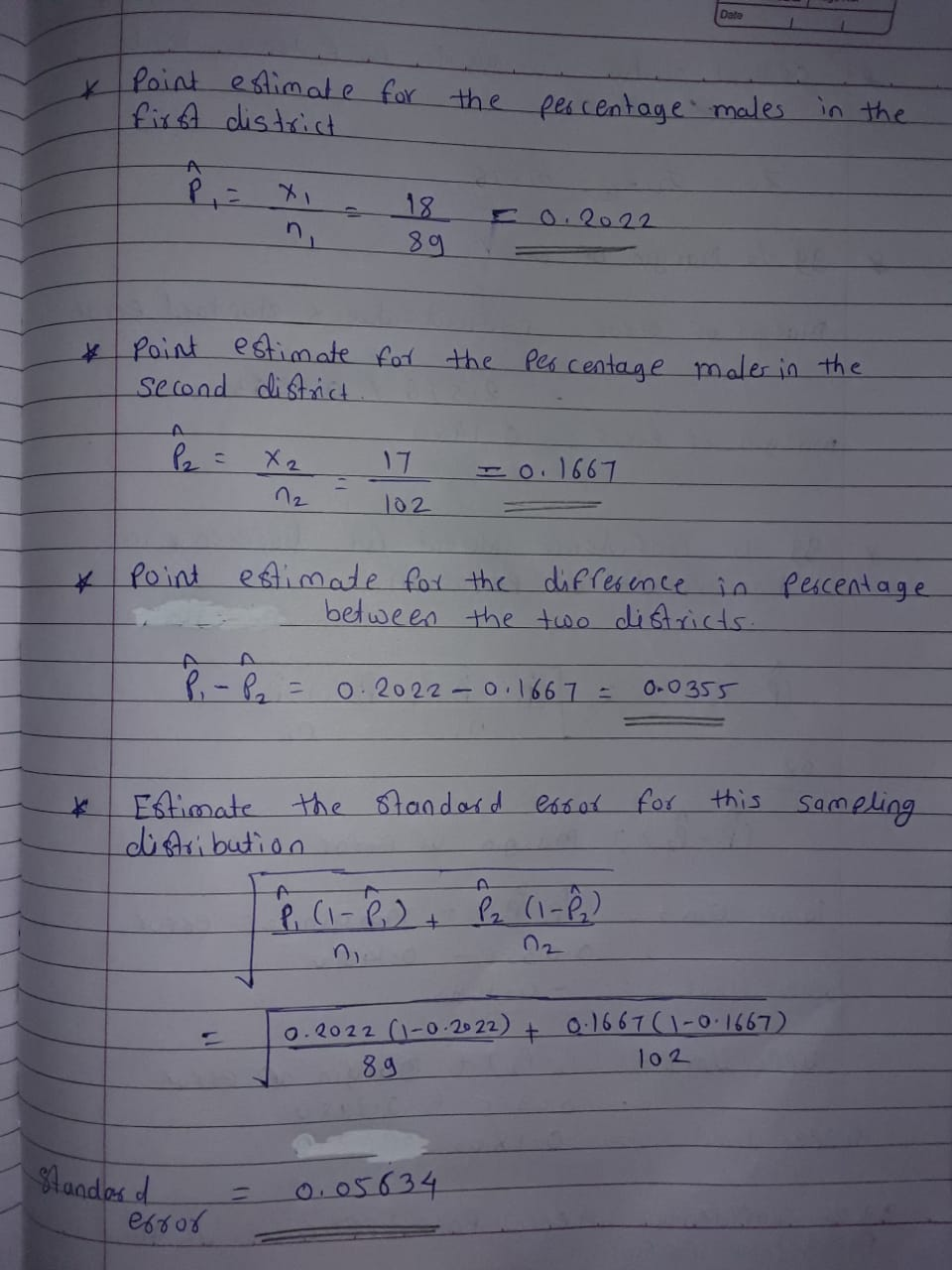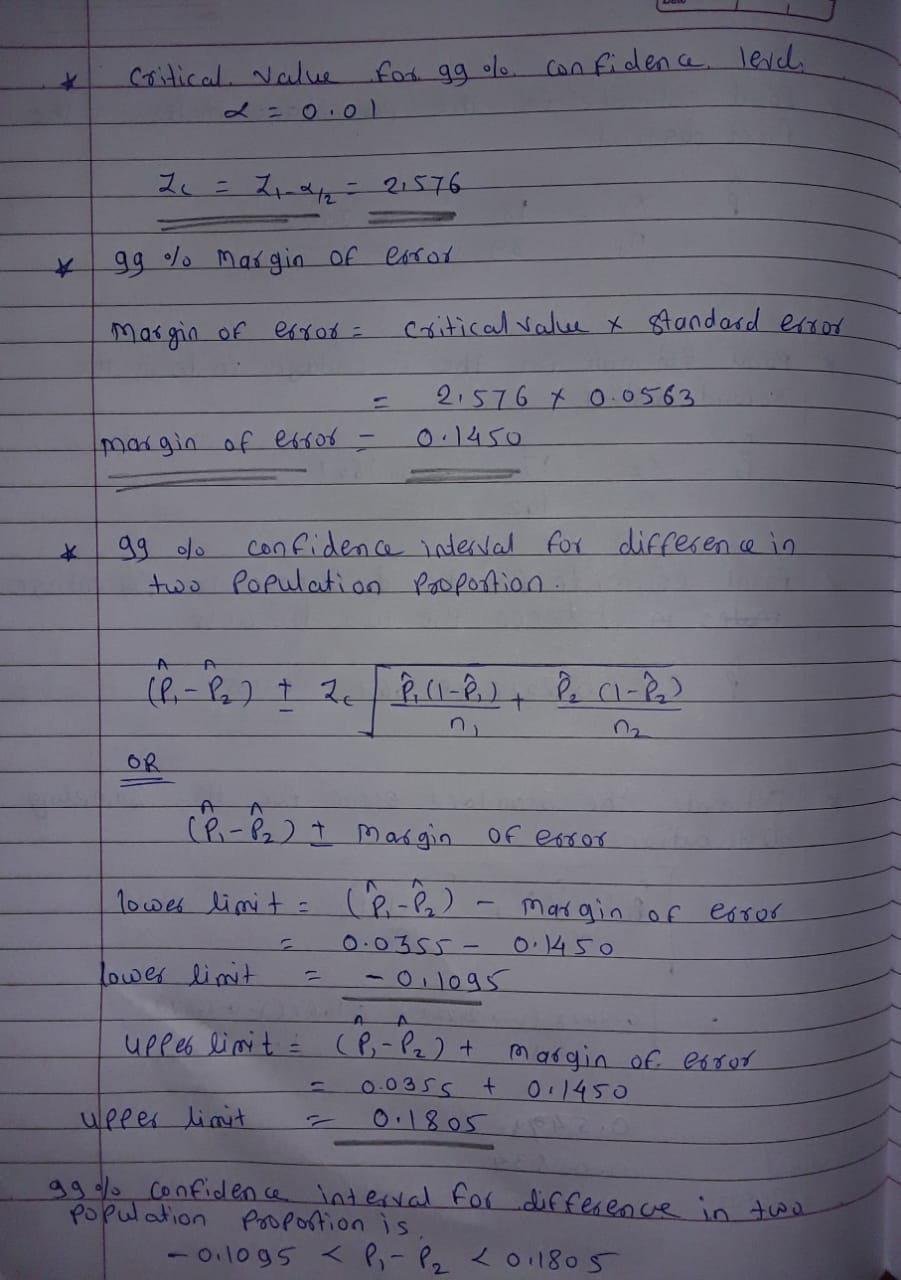##### Question

In: Statistics and Probability

# In one school district, there are 89 elementary school (K-5) teachers, of which 18 are male (or male-identifying).

In one school district, there are 89 elementary school (K-5) teachers, of which 18 are male (or male-identifying). In a neighboring school district, there are 102 elementary teachers, of which 17 are male. A policy researcher would like to calculate the 99% confidence interval for the difference in proportions of male teachers.

To keep the signs consistent for this problem, we will calculate all differences as p1−p2. That is, start with the percentage from the first school district and then subtract the percentage from the second district. Failing to do so may end up with “correct” answers being marked as wrong.

Point estimate for the percentage males in the first district:
ˆp1=
Point estimate for the percentage males in the second district:
ˆp2=
Point estimate for the difference in percentages between the two districts:
ˆp1−ˆp2=

Estimate of the standard error for this sampling distribution (distribution of differences):
√ˆp1(1−ˆp1)n1+ˆp2(1−ˆp2)n2=

Critical value for the 99% confidence level:
zc.v.=

99% margin of error:
M.E.=

99% confidence interval:

≤p1−p2≤

## Solutions

##### Expert Solution## Related Solutions

##### Salaries for teachers in a particular elementary school district are normally distributed with a mean of...
Salaries for teachers in a particular elementary school district are normally distributed with a mean of $44,000 and a standard deviation of$6,500. We randomly survey ten teachers from that district. 1.Find the probability that the teachers earn a total of over $400,000 2.If we surveyed 70 teachers instead of ten, graphically, how would that change the distribution in part d? 3.If each of the 70 teachers received a$3,000 raise, graphically, how would that change the distribution in part...
##### Salaries for teachers in a particular elementary school district are normally distributed with a mean of...
Salaries for teachers in a particular elementary school district are normally distributed with a mean of $44,000 and a standard deviation of$6,500. We randomly survey ten teachers from that district. Find the 85th percentile for the sum of the sampled teacher's salaries to 2 decimal places.
##### Within a school district, students were randomly assigned to one of two Math teachers - Mrs....
Within a school district, students were randomly assigned to one of two Math teachers - Mrs. Smith and Mrs. Jones. After the assignment, Mrs. Smith had 30 students, and Mrs. Jones had 25 students. At the end of the year, each class took the same standardized test. Mrs. Smith's students had an average test score of 78, with a standard deviation of 10; and Mrs. Jones' students had an average test score of 85, with a standard deviation of 15....
##### A researcher claims that the mean of the salaries of elementary school teachers is greater than...
A researcher claims that the mean of the salaries of elementary school teachers is greater than the mean of the salaries of secondary school teachers in a large school district. The mean of the salaries of a random sample of 26 elementary school teachers is $48,250 and the sample standard deviation is$3900. The mean of the salaries of 24 randomly selected secondary school teachers is $45,630 with a sample standard deviation of$5530. At ? = 0.05, can it...
##### A study was conducted to estimate the difference in the mean salaries of elementary school teachers...
A study was conducted to estimate the difference in the mean salaries of elementary school teachers from two neighboring states. A sample of 10 teachers from the Indiana had a mean salary of $28,900 with a standard deviation of$2300. A sample of 14 teachers from Michigan had a mean salary of $30,300 with a standard deviation of$2100. Determine a 95% confidence interval for the difference between the mean salary in Indiana and Michigan.(Assume population variances are different.) *Include...